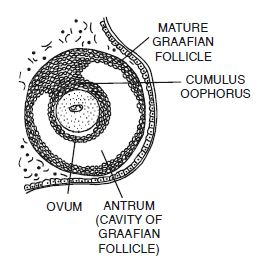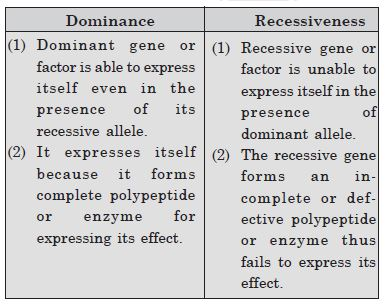# NCERT Solutions for Class 11 Chemistry Chapter 1 - Some Basic Concepts of Chemistry

##### Question 1:

Calculate the molecular mass of the following : (i) H2O (ii) CO2 (iii) CH4

(i) Molecular mass of H2O = 2 × 1 + 16
= 2 + 16
= 18 amu
(ii) Molecular mass of CO2 = 12 + 2 × 16
= 12 + 32
= 44 amu
(iii) Molecular mass of CH4=12 + 4 × 1
= 12 + 4
= 16 amu

##### Question 2:

Calculate the mass per cent of different elements present in sodium sulphate (Na2SO4).

LMolecular mass of sodium sulphate (Na2SO4)
= 2 × 23 + 32 + 4 × 16
= 46 + 32 + 64
= 142

##### Question 3:

Determine the empirical formula of an oxide of iron which has 69.9% iron and 30.1% dioxygen by mass.

Fe = 69.9%, O = 30.1%

##### Question 4:

Calculate the amount of carbon dioxide that could be produced when
(i) 1 mole of carbon is burnt in air.
(ii) 1 mole of carbon is burnt in 16 g of dioxygen.
(iii) 2 moles of carbon are burnt in 16 g of dioxygen.

1 mole 1 mole 1 mole (44 g)
1 mole of C will produce 44 g of CO2
12 g 32 g 44 g
(Limiting reactant)
32 g of oxygen reacts with carbon to produce
CO2 = 44 g
1 mole 1 mole 1 mole
12 g 32 g 44 g
(Limiting reactant)
32 g of oxygen produces CO2 = 44 g

##### Question 5:

Calculate the mass of sodium acetate (CH3COONa) required to make 500 mL of 0.375 molar aqueous solution. Molar mass of sodium acetate is 82.0245 g mol–1.

Molar mass of sodium acetate = 82.0245 g/mol
Mass of sodium acetate required to make 500 ml of 0.375 M solution.

= 15.38 g.

##### Question 6:

Calculate the concentration of nitric acid in moles per litre in a sample which has a density, 1.41 g mL–1 and the mass per cent of nitric acid in it being 69%.

100 g of solution of HNO3 contains
HNO3 = 69 g

##### Question 7:

How much copper can be obtained from 100 g of copper sulphate (CuSO4) ?

##### Question 8:

Determine the molecular formula of an oxide of iron in which the mass per cent of iron and oxygen are 69.9 and 30.1 respectively. Molecular mass is 160 amu.

Empirical Formula

##### Question 9:

Calculate the atomic mass (average) of chlorine using the following data :Average atomic mass##### Question 10:

In three moles of ethane (C2H6),
calculate the following :
(i) Number of moles of carbon atoms.
(ii) Number of moles of hydrogen atoms.
(iii) Number of molecules of ethane.

Ethane has the molecular formula = C2H6 (i) 1 mole of ethane contains C atoms
= 2 moles
3 moles of ethane contains C atoms
= 6 moles
(ii) 1 mole of ethane contains H atoms
= 6 moles
3 moles of ethane contains H atoms
= 18 moles
(iii) 1 mole of ethane contains molecules
= 6.022 × 1023
3 mole of ethane contains molecules

##### Question 11:

What is the concentration of sugar (C12H22O11) in mol L–1 if its 20 g are dissolved in enough water to make a final volume up to 2 L ?

##### Question 12:

If the density of methanol is 0.793 kg L–1, what is its volume needed for making 2.5 L its 0.25 M solution ?

Density of methanol = 0.793 kg L–1
Molecular mass of methanol (CH3OH)
= 12 + 3 + 16 + 1 = 32
2.5 L of 0.25 M methanol solution contains
methanol = 2.5 × 0.25 × 32
= 20 g
= 0.020 kg
0.793 kg of methanol is present in volume
= 1000 ml
0.020 kg of methanol is present in volume

= 25.22 ml = 0.02522 L.

##### Question 13:

Pressure is determined as force per unit area of the surface. The SI unit of pressure, pascal is as shown below : 1Pa = 1 N m–2 If mass of air at sea level is 1034 g cm–2, calculate the pressure in pascal.

Mass of air at sea level = 1034 g/cm2 Acceleration due to gravity, g = 9.8 ms2

##### Question 14:

What is the SI unit of mass ? How is it defined ?

S.I. unit of mass is kilogram and it is equal to the mass of the international prototype of the kilogram.

Or

It is defined as the mass of Pt-Ir cylinder that is stored in an air tight jar at the International Bureau of weights and Measures in France.

##### Question 15:

Match the following prefixes with their multiples :

##### Question 16:

What do you mean by significant figures ?

Significant figures refer to the number of digits in a number which has some importance in the magnitude of a given number e.g. 30.4570 has no. of significant figures = 5.

##### Question 17:

A sample of drinking water was found to be severely contaminated with chloroform, CHCl3, supposed to be carcinogen. The level of contamination was 15 ppm (by mass)
(i) Express this in per cent by mass.
(ii) Determine the molality of
chloroform in the water sample.

##### Question 18:

Express the following in the scientific notation :
(i) 0.0048 (ii) 234,000
(iii) 8008 (iv) 500.0
(v) 6.0012

(i) 4.8 × 10–3
(ii) 2.34 × 105
(iii) 8.008 × 103
(iv) 5.000 × 102
(v) 6.0012 × 10–0.

##### Question 19:

How many significant figures are present in the following ? (i) 0.0025 (ii) 208
(iii) 5005 (iv) 126,000
(v) 500.0 (vi) 2.0034

(i) Two
(ii) Three
(iii) Four
(iv) Three
(v) Four
(vi) Five

##### Question 20:

Round up the following upto three significant figures :
(i) 34.216 (ii) 10.4107
(iii) 0.04597 (iv) 2808

(i) 34.2
(ii) 10.4
(iii) 0.0460
(iv) 2810.

##### Question 21:

The following data are obtained when dinitrogen and dioxygen react together to form different compounds :
Mass of dinitrogen Mass of dioxygen
(i) 14 g 16 g
(ii) 14 g 32 g
(iii) 28 g 32 g
(iv) 28 g 80 g
(a) Which law of chemical combination is obeyed by the above experimental data ? Given its statement.
(b) Fill in the blanks in the following conversions :
(i) 1 km =.............. mm = ................. pm
(ii) 1 mg =............... kg = ................... ng
(iii) 1 mL =.............. L = ................. dm3.

(a) Law of Multiple proportions. It states that if two elements combine to form two or more compounds, the weights of one of the elements which combine with a fixed weight of another bear a simple whole number ratio to one another.

##### Question 22:

If the speed of light is 3.0 × 108 m s–1, calculate the distance covered by light in 2.00 ns.

##### Question 23:

In a reaction

Identify the limiting reagent, if any, in
the following reaction mixtures :
(i) 300 atoms of A + 200 molecules of B
(ii) 2 mol A + 3 mol B
(iii) 100 atoms of A + 100 molecules of B
(iv) 5 mol A + 2.5 mol B
(v) 2.5 mol A + 5 mol B.

(i) B
(ii) A
(iii) No, because it is a stoichiometric mixture
(iv) B
(v) A.

##### Question 24:

Dinitrogen and dihydrogen react with each other produce ammonia according to the following chemical equation :

(i) Calculate the mass of ammonia
produced if 2.00 × 103 g dinitrogen reacts with 1.00 × 103 g of dihydrogen. (ii) Will any of the two reactants remain unreacted ?
(iii) If yes, which one and what would be its mass ?

##### Question 25:

How are 0.50 mol Na2CO3 and 0.50 M Na2CO3 different ?

0.5 mol Na2CO3 represents 0.5 mol of solid Na2CO3 0.50 M Na2CO3 represents 0.50 molar solution of Na2CO3 i.e. 0.50 mol of Na2CO3 is present per litre of the solution.

##### Question 25:

How are 0.50 mol Na2CO3 and 0.50 M Na2CO3 different ?

0.5 mol Na2CO3 represents 0.5 mol of solid Na2CO3 0.50 M Na2CO3 represents 0.50 molar solution of Na2CO3 i.e. 0.50 mol of Na2CO3 is present per litre of the solution.

##### Question 25:

If ten volumes of dihydrogen gas reacts with five volumes of dioxygen gas, how many volumes of water vapour would be produced ?

##### Question 26:

Convert the following into basic units :
(i) 28.7 pm
(ii) 15.15 pm
(iii) 25365 mg.

(i) 28.7 pm = 28.7 × 10 –12 m
= 2.87 × 10 –11 m
(ii) 15.15 pm = 15.15 × 10 –12 m
= 1.515 × 10 –11 m

##### Question 27:

Which one of the following will have largest number of atoms ? (i) 1 g Au (s)
(ii) 1 g Na (s)
(iii) 1 g Li (s)
(iv) 1 g of Cl2 (g).

##### Question 28:

Calculate the molarity of a solution of ethanol in water in which the mole fraction of ethanol is 0.040.

Mole fraction of ethanol = 0.04
Let the solution is dilute and its molality = m
Molality is m,

##### Question 29:

What will be the mass of one 12C atom in g ?

##### Question 30:

How many significant figures should be present in the answer of the following calculations ?

(ii) 5 × 5.364
(iii) 0.0125 + 0.7864 + 0.0215.

(i) No. of significant figures = Three
(ii) No. of significant figures = Four
(iii) No. of significant figures = Four.

##### Question 31:

Use the data given in the following table to calculate the molar mass of naturally occurring argon :

##### Question 32:

Calculate the number of atoms in each of the following (i) 52 moles of Ar (ii) 52 u of He (iii) 52 g of He.

Atomic mass of He = 4u
(i) No. of atoms in 52 moles of Ar
= 52 × 6.022 × 1023
= 313.144 × 1023
= 3.13144 × 1025

##### Question 33:

A welding fuel gas contains carbon and hydrogen only. Burning a small sample of it in oxygen gives 3.38 g carbon dioxide, 0.690 g of water and no other products. A volume of 10.0 L (measured at STP) of this welding gas is found to weigh 11.6 g. Calculate (i) empirical formula (ii) molar mass of the gas, and (iii) molecular formula.